# Coding Logistic Regression from Scratch

## Implementing Logistic Regression from absolute scratch using python.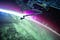Photo by NASA on Unsplash

This post follows the logistic regression post in the “Basics and Beyond” series. So if you are new to machine learning then I would recommend going through that post first but if you already know what logistic regression is then let's get to work!

In this post, we will be coding a logistic regression model from the very basics using python. So let's get started!

# Data

At the core of any machine learning algorithm is data. Your machine learning models will always need data to “learn” from. …

# Coding Linear Regression from Scratch

## Implementing Linear Regression from absolute scratch using python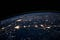Photo by NASA on Unsplash

This post follows the linear regression post in the ‘Basics and Beyond’ series so if you are just getting started with machine learning I would recommend going through that post first and then starting with this tutorial. If you already have an idea about what linear regression is then lets get started!

In this post we will be coding the entire linear regression algorithm from absolute scratch using python so we will really be getting our hands dirty today!

Let's go!

# Data

The first step for any machine learning problem is getting the data. There is no machine “learning” if there…

# Basics and Beyond: Logistic Regression

## Taking apart the logistic regression algorithm piece by piece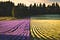Photo by Dan Meyers

This post will walk you through logistic regression from the very basics. In order to master machine learning it is imperative to have the basics very clear. It may seem exhausting at first but once you have the basics perfect, writing the code will be a cake walk. Most posts try to cover everything in one go and that honestly becomes overwhelming at times. Trying to understand complex equations and then switching over to code then back to the mathematics just makes it difficult to keep up. Well this series aims to guide you through machine learning with a slightly…

# Basics and Beyond: Linear Regression

## Taking apart the linear regression algorithm piece by piece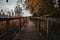Photo by Matthias Oberholzer

This post will walk you through linear regression from the very basics. When starting off with machine learning Linear Regression is probably one of the first topics that one comes across and honestly it is one of the most widely and easily implemented algorithms as well. In order to master machine learning it is imperative to have the basics very clear. It may seem exhausting at first but once you have the basics perfect, writing the code will be a cake walk. Most posts try to cover everything in one go and that honestly becomes overwhelming at times. Trying to…

# Basics and Beyond: Gradient Descent

## Decoding gradient descent step by step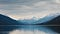Photo by Jake Hills

This post aims to take you from the very basics to advanced concepts in gradient descent. When starting off with machine learning, Gradient Descent is probably one of the initial concepts one comes across. Let’s get started!

All machine learning algorithms require an optimization algorithm and Gradient Descent is just that. It is an optimization algorithm that aims to adjust parameters in order to minimize the cost function .## Kumud Lakara

Crazy about everything AI! Researching in areas of Machine Learning, Deep Learning and Reinforcement Learning.